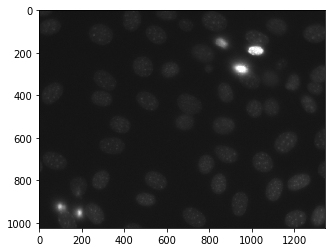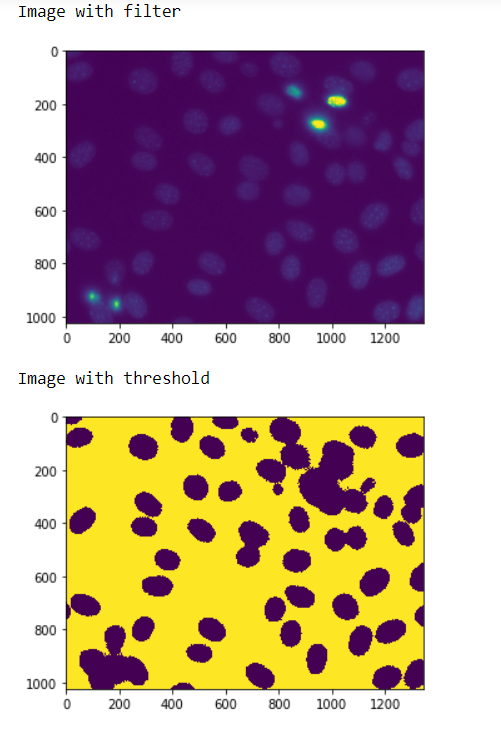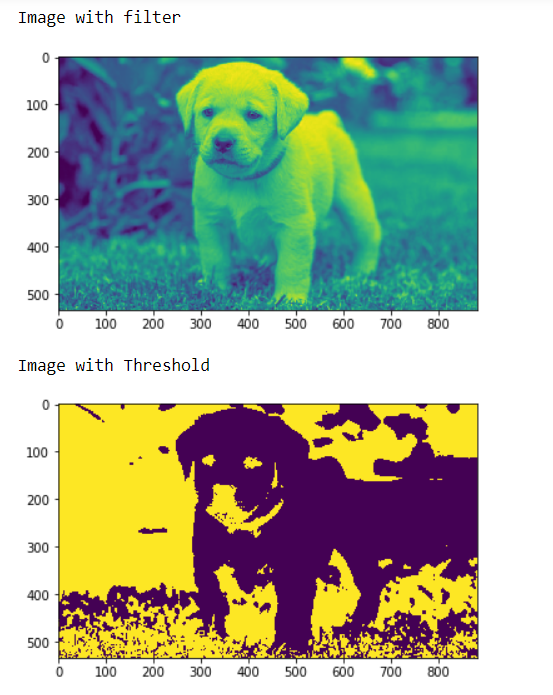# Mahotas – Setting Threshold

In this article we will see how we can set threshold to the images in mahotas. For this we are going to use the fluorescent microscopy image from a nuclear segmentation benchmark. We can get the image with the help of command given below

`mhotas.demos.nuclear_image()`

Image thresholding is a simple form of image segmentation. It is a way to create a binary image from a grayscale or full-color image. This is typically done in order to separate “object” or foreground pixels from background pixels to aid in image processing.

Below is the nuclear_imageIn order to set threshold to the image we will take the image object which is numpy.ndarray and will divide the array with the threshold value, here threshold value is the means value, below is the command to do this

`img = (img < img.mean())]`

Example 1 :

 `# importing required libraries ` `import` `mahotas as mh ` `import` `mahotas.demos ` `import` `numpy as np ` `from` `pylab ``import` `imshow, show ` ` `  `# getting nuclear image ` `nuclear ``=` `mh.demos.nuclear_image() ` ` `  ` `  `# filtering the image ` `nuclear ``=` `nuclear[:, :, ``0``] ` ` `  `print``(``"Image with filter"``) ` `# showing the image ` `imshow(nuclear) ` `show() ` ` `  `# setting image threshold ` `nuclear ``=` `(nuclear < nuclear.mean()) ` ` `  `print``(``"Image with threshold"``) ` `# showing the threshold image ` `imshow(nuclear) ` `show() `

Output :Example 2 :

 `# importing required libraries ` `import` `numpy as np ` `import` `mahotas ` `from` `pylab ``import` `imshow, show ` `  `  `# loading iamge ` `img ``=` `mahotas.imread(``'dog_image.png'``) ` `   `  `# filtering the imagwe ` `img ``=` `img[:, :, ``0``] ` `    `  `print``(``"Image with filter"``) ` `# showing the image ` `imshow(img) ` `show() ` `  `  `# setting threshold ` `img ``=` `(img < img.mean()) ` `  `  `print``(``"Image with Threshold"``) ` `# showing the threshold image ` `imshow(img) ` `show() `

Output :Attention geek! Strengthen your foundations with the Python Programming Foundation Course and learn the basics.

To begin with, your interview preparations Enhance your Data Structures concepts with the Python DS Course.

My Personal Notes arrow_drop_upCheck out this Author's contributed articles.

If you like GeeksforGeeks and would like to contribute, you can also write an article using contribute.geeksforgeeks.org or mail your article to contribute@geeksforgeeks.org. See your article appearing on the GeeksforGeeks main page and help other Geeks.

Please Improve this article if you find anything incorrect by clicking on the "Improve Article" button below.

Article Tags :

Be the First to upvote.

Please write to us at contribute@geeksforgeeks.org to report any issue with the above content.